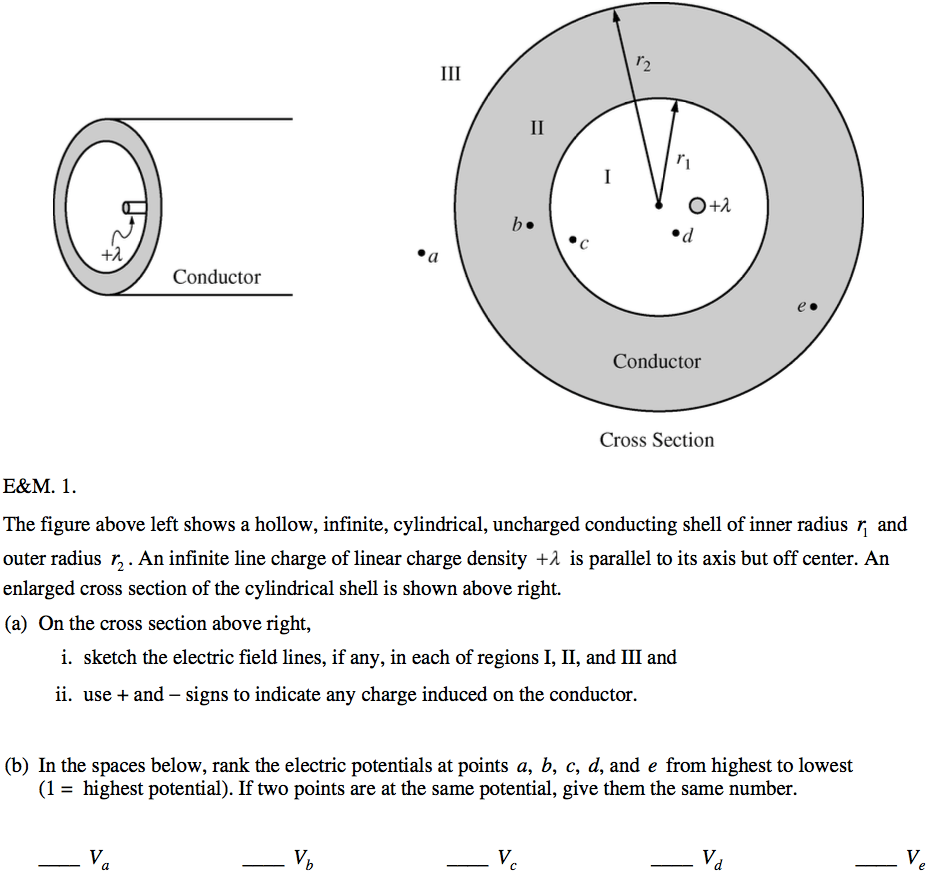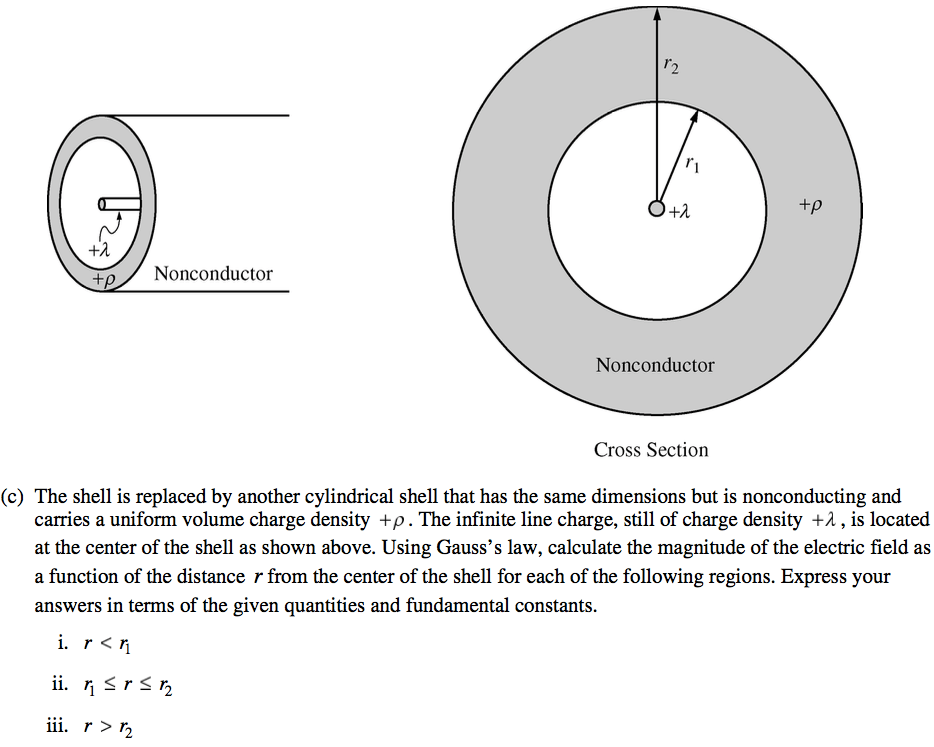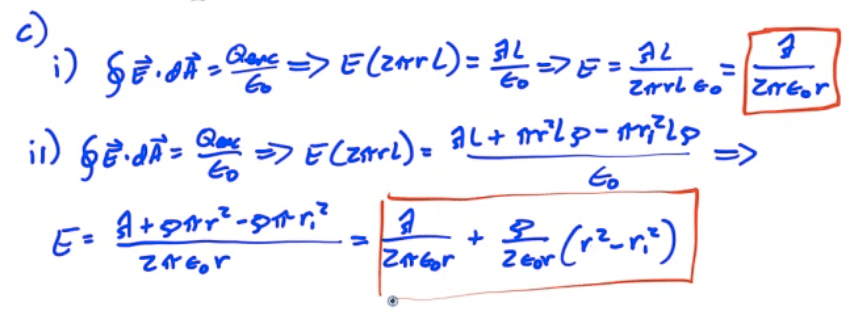# Charges in a Conductor

• Charge is free to move until the E=0• All charge resides at surface• Field lines are perpendicular to the surface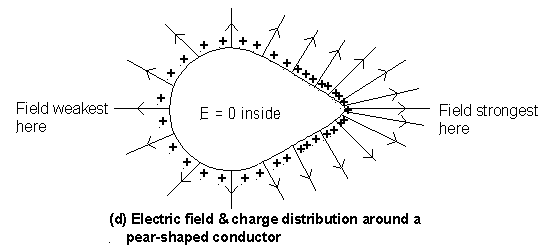# Electric Field at the Surface of a Conductor

•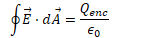•••• E increases as r σ increases.

# Hollow Conductors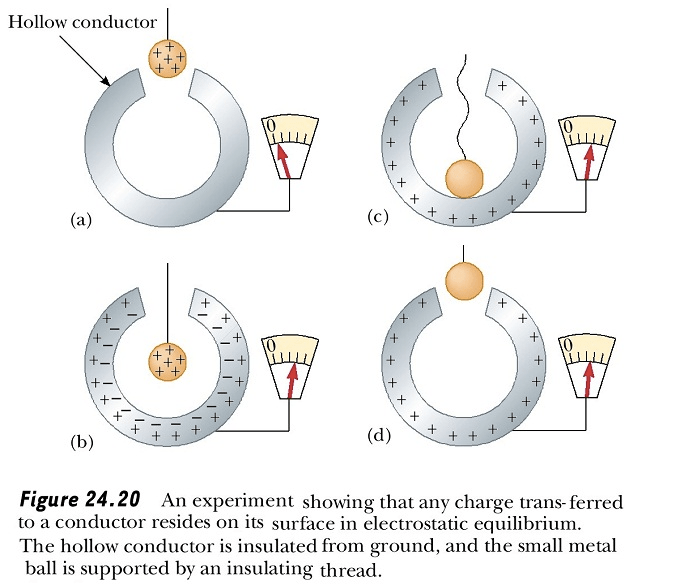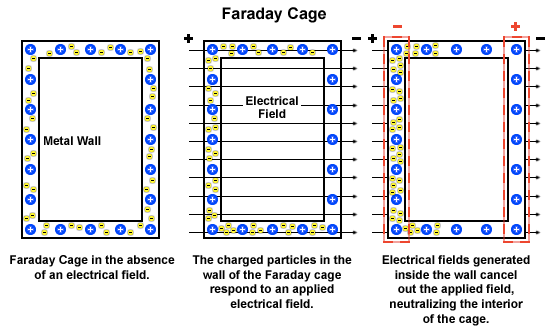# Example 1: Conducting Spheres Connected by a Wire• Two conducting spheres, A and B, are placed a large distance from each other. The radius of Sphere A is 5 cm, and the radius of Sphere B is 20 cm. A charge Q of 200 nC is placed on Sphere A, while Sphere B is uncharged. The spheres are then connected by a wire. Calculate the charge on each sphere after the wire is connected

•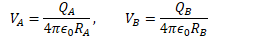••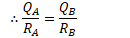•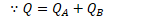••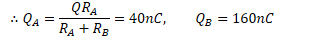# 2004 Free Response Question